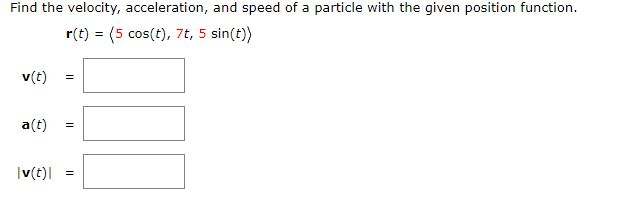# Find the velocity, acceleration, and speed of a particle with the given position function. r(t) = (5 cos(t), 7t, 5 sin(t)) v(t) a(t) |v(t) = II II

Questionhelp_outlineImage TranscriptioncloseFind the velocity, acceleration, and speed of a particle with the given position function. r(t) = (5 cos(t), 7t, 5 sin(t)) v(t) a(t) |v(t) = II II fullscreen

### Want to see this answer and more?

Experts are waiting 24/7 to provide step-by-step solutions in as fast as 30 minutes!*

*Response times may vary by subject and question complexity. Median response time is 34 minutes for paid subscribers and may be longer for promotional offers.
Tagged in
Math
Calculus

### Other